• 傅里叶变换完成的功能
2021-05-20 11:22:27

变换常常可以简化问题的分析和求解过程。人们常会在这样那样的场合使用这一技巧。在科学研究的许多领域,人们发现傅里叶变换(FT)对于问题的求解和简化特别有用。

傅里叶变换方法又称为谱分析方法，具有普适性。例如线性系统输出的傅里叶变换是输入信号和系统响应函数傅里叶变换的乘积。天线的方向图是其发射电流的傅里叶变换。在光学系统中，会聚透镜的前焦和后焦面上的振幅分布存在傅里叶变换关系。一个随机过程的功率谱密度由该过程的自相关函数的傅里叶变换确定。许多常微分方程和偏微分方程的解可利用傅里叶变换求得。这些截然不同领域的有关问题可以通过傅里叶变换联系在一起。

傅里叶变换可以看作是时间域上的函数在频率域上的表示。而且其傅里叶变换谱函数在频率域中包含的信息和原时间域函数所包含的信息是等价的，不同的仅是信息的表述方式。谱分析方法的基本思想来源于经典的Ritz-Galerkin方法。由于许许多多问题都可应用傅里叶变换，因此计算数学很自然地将傅里叶变换离散化产生所谓离散傅里叶变换(DFT)，然后使用计算机求解。当然这时需要研究连续傅里叶变换和离散傅里叶变换的关系，需要研究离散傅里叶变换的性质并寻求离散傅里叶变换好的快速计算方法。

在谱分析方法中，大多数情况下原函数不是局部支集的，所以谱函数在每一点上的值都与原函数在所有点上的值有联系，因此由逼近方法导出的代数方程组中系数矩阵基本上是满的，求解计算量太大。进一步分析发现求解一个N点数据离散傅里叶变换的复数乘法计算量正比于N2。为了减少离散误差，必须取很大的N。但当N很大，即便使用高速计算机，其运算时间还是太长，是不可实现的。因此在相当长的时间内，傅里叶变换方法没有得到应有的重视、发展和应用。

人们不懈地寻找减少离散傅里叶变换计算量的方法和技术。直至1965年，Cooley-Tukey发表的算法，才得到人们认同。人们称之为“快速傅里叶变换(FFT)”方法。对于长度为N的复序列，它的复数乘法计算量为O(Nlog2N)，因此计算量的节省是巨大的。更值得重视的是对于多维问题，其快速傅里叶变换复数乘法计算量并不按数据点数指数形式增加。例如对于N×N×N个点的三维离散傅里叶变换，数据点数为N3，其快速傅里叶变换复数乘法计算量为O(3N3log2N)=O(N3log2N3)。总之FFT算法有效地解决了傅里叶变换计算量太大的问题。FFT算法的出现使得傅里叶变换的研究和应用的面貌出现根本转变。人们开始重新考虑它的优点，越来越多地将它用于解决各种各样的实际问题。例如在雷达、声纳、地震勘探、通信、医疗、气象、射电天文学等领域FFT算法都得到了广泛的应用。目前FFT算法仍处于蓬勃发展的状态，与之相应的计算数学的这个重要分支也处于迅速发展之中。

本书共分5章。第1章首先讨论周期函数傅里叶级数，傅里叶积分及其收敛性。以此为基础讨论傅里叶变换的定义和存在条件、傅里叶变换的实例、广义函数的傅里叶变换。傅里叶变换的性质、对称关系和傅里叶变换的常用形式。

第2章首先介绍离散时间序列的傅里叶变换，进而讨论离散傅里叶变换的定义和性质。具体讨论实序列的离散傅里叶变换的性质、离散正弦变换和离散余弦变换，离散傅里叶级数及其最佳平方逼近。最后讨论频谱混叠和频谱渗漏问题，给出连续和离散傅里叶变换之间的关系。

第3章讨论离散傅里叶变换快速算法的基本原理，给出复序列基2算法及其相关程序。该算法和程序构成本书FFT算法的核心和基础。进而利用FFT程序给出了实序列的傅里叶变换、离散正弦变换、离散余弦变换，傅里叶级数、谱函数近似计算、功率谱估计等问题的快速算法和相关程序。

第4章讨论一维卷积及其性质和物理意义。离散卷积的定义，离散卷积定理，离散卷积和连续卷积的关系。给出利用FFT算法和程序求卷积和解卷积的快速算法和实用程序。本章还讨论相关、离散相关和离散相关定理，并利用FFT算法和程序给出离散相关的快速算法和实用程序。

第5章主要讨论多维傅里叶变换问题，给出二维、三维和n维傅里叶变换定义及其性质。具体讨论二维复序列2D-DFT的行列算法和二维实序列2D-DFT的行列算法、存储技术及其相关程序。对于三维复序列的3D-DFT给出2D-DFT行列算法的一个推广及其相关程序。对三维实序列3D-DFT给出了降维算法及其相关程序。这两种3D-DFT的快速算法皆可方便地推广应用于更高维的傅里叶变换的快速计算问题。

总之,本书给出了傅里叶变换的数学基础，离散傅里叶变换和连续傅里叶变换的关系。各种和傅里叶变换相关问题的快速算法及其具体实现C语言程序。在编程中，一般将C语言程序分为两部分，即一个包含主函数的文件和一个或几个包含功能子函数的文件。主函数文件主要是设置参数，提供初始数据，调用功能子函数和输出计算结果。读者根据实际问题和需要，可参照书中的实例对主函数作必要的修改。功能子函数主要具体实现算法，可直接调用。本书程序皆用Visual C++6.0调试通过。由于例题计算结果的数据量太大，数据文件皆不收入本书。

本书由蒋长锦和电子科学与技术系2002级硕士生蒋勇合作完成。前者负责组稿和撰写，后者完成程序的编制和调试。

作者感谢中国科学技术大学教务处、出版社、理学院和数学系等部门对本书编写的关心和支持。感谢李洪梅、蒋智同志为排版付出的辛勤劳动。感谢夫人吴康平为保证作者全身心投入写作作出的无私奉献。

限于作者水平，本书难免仍存在不妥和错误，恳切希望读者批评、指正和谅解。

作者

2004年6月于中国科学技术大学

更多相关内容
• [Python图像处理六] :Opencv图像傅里叶变换傅里叶变换原理及实现 一、傅里叶变换 1、傅里叶变换原理 2、自定义傅里叶变换功能函数 3、OpenCV库函数实现傅里叶变换 二、傅里叶变换 1、傅里叶变换原理 2、...

本专栏主要介绍如果通过OpenCv-Python进行图像处理，通过原理理解OpenCv-Python的函数处理原型，在具体情况中，针对不同的图像进行不同等级的、不同方法的处理，以达到对图像进行去噪、锐化等一系列的操作。同时，希望观看本专栏的小伙伴可以理解到OpenCv进行图像处理的强大哦，如有转载，请注明出处(原文链接和作者署名)，感谢各位小伙伴啦！

上次博客，我们讲解了OpenCV图像去噪的几种基本方法，包括均值滤波、中值滤波、高斯滤波等，也就是在图像时域上进行去噪的方法，其中，高斯滤波和中值滤波是需要重点掌握的图像去噪方法，因为对图像的高斯噪声和椒盐噪声去除的效果比较好；除了以上时域上的去噪方法之外，OpenCV也提供了对图像频域上的去噪的函数方法，例如高通滤波和低通滤波，通常用作图像除噪、图像增强和锐化等，因此，高通滤波和低通滤波也是我们学习OpenCV需要掌握的两种图像去噪的方法，但由于高通和低通滤波的实现原理是通过傅里叶变换后进行处理，然后通过傅里叶逆变换进行实现的，所以首先我们需要了解图像傅里叶变换和傅里叶逆变换的原理。

本次博客，林君学长将带大家了解OpenCV图像的傅里叶变换和逆变换原理，通过原理编写傅里叶变换和逆变换的功能函数，并同时介绍OpenCV傅里叶变换和逆变换的库函数使用方法，一起学习吧！

在进行傅里叶变换学习时，我们必须要明白为什么要在频率域研究图像增强？

• 可以利用频率成分和图像外表之间的对应关系。一些在空间域表述困难的增强任务，在频率域中变得非常普通
• 滤波在频率域更为直观，它可以解释空间域滤波的某些性质
• 可以在频率域指定滤波器，做反变换，然后在空间域使用结果滤波器作为空间域滤波器的指导
• 一旦通过频率域试验选择了空间滤波，通常实施都在空间域进行

提示：傅里叶变换是为后面低通滤波或者说是高通滤波做准备哦，一般来说，处理的是灰度图像，通过上面的介绍我们也可以看出，但下面的原理我们通过处理彩色图像编写的，而OpenCV库函数是在处理灰度图像基础上实现的

# 一、傅里叶变换

• 傅里叶变换（Fourier Transform，FT）后，对同一事物的观看角度随之改变，可以从频域里发现一些从时域里不易察觉的特征。某些在时域内不好处理的地方，在频域内可以容易地处理。

## 1、傅里叶变换原理

1）、什么是傅里叶变换？
能将满足一定条件的某个函数表示成三角函数（正弦和/或余弦函数）或者它们的积分的线性组合。在不同的研究领域，傅立叶变换具有多种不同的变体形式，如连续傅立叶变换和离散傅立叶变换。傅里叶变换是一种分析信号的方法，它可分析信号的成分，也可用这些成分合成信号。许多波形可作为信号的成分，比如正弦波、方波、锯齿波等，傅里叶变换用正弦波作为信号的成分。
傅里叶变换的实质是将一个信号分离为无穷多多正弦/复指数信号的加成，也就是说，把信号变成正弦信号相加的形式——既然是无穷多个信号相加，那对于非周期信号来说，每个信号的加权应该都是零——但有密度上的差别，你可以对比概率论中的概率密度来思考一下——落到每一个点的概率都是无限小，但这些无限小是有差别的所以，傅里叶变换之后，横坐标即为分离出的正弦信号的频率，纵坐标对应的是加权密度。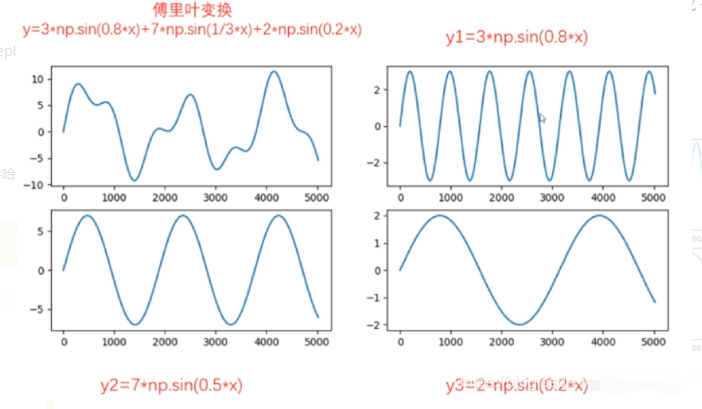2）、傅里叶变换作用？
傅里叶变换可以将一个时域信号转换成在不同频率下对应的振幅及相位，其频谱就是时域信号在频域下的表现，而反傅里叶变换可以将频谱再转换回时域的信号。最简单最直接的应用就是时频域转换。将图像时域处理上面复杂的特征转为频域实现简单化，对简单化的特征进行处理，然后再通过傅里叶逆变换回到原来的图像，如下所示：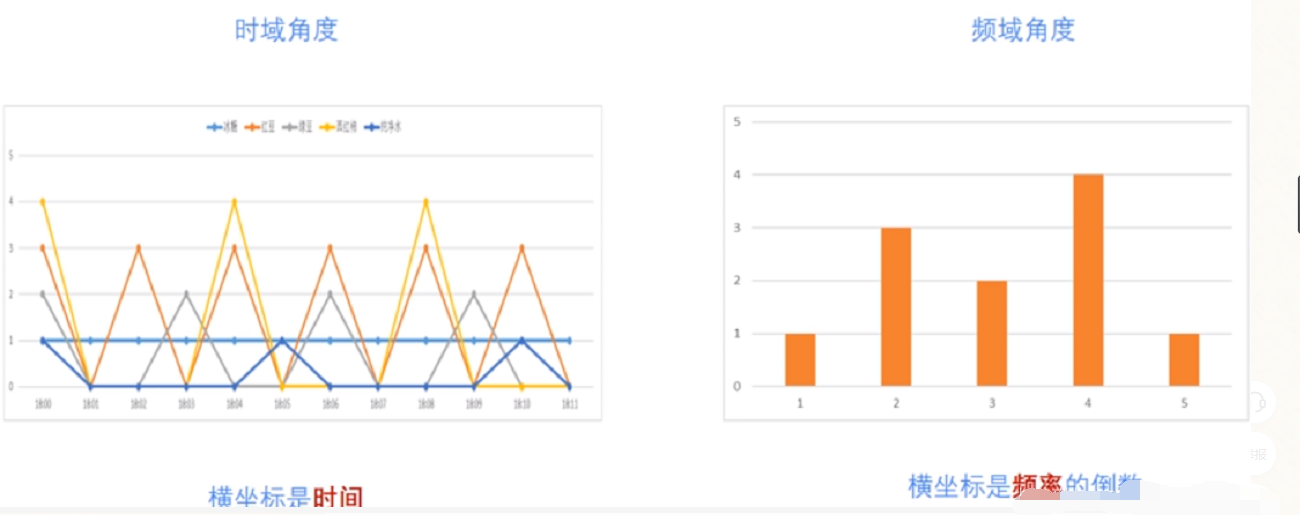3）、图像进行傅里叶变换原理
我们知道，灰度图像是由二维的离散的点构成的。二维离散傅里叶变换（Two-Dimensional Discrete Fourier Transform）常用于图像处理中，对图像进行傅里叶变换后得到其频谱图。频谱图中频率高低表征图像中灰度变化的剧烈程度。图像中边缘和噪声往往是高频信号，而图像背景往往是低频信号。我们在频率域内可以很方便地对图像的高频或低频信息进行操作，完成图像去噪，图像增强，图像边缘提取等操作，因此，对图像进行傅里叶变换的原理如下公式：
F ( u , v ) = ∑ x = 0 H − 1 ∑ y = 0 W − 1 f ( x , y ) e − 2 j π ( u x / H + v y / W ) 图 像 高 H ， 宽 W 。 F ( u , v ) 表 示 频 域 图 像 ， f ( x , y ) 表 示 时 域 图 像 。 u 的 范 围 为 [ 0 , H − 1 ] ， v 的 范 围 为 [ 0 , W − 1 ] ↑ F(u,v)=\sum_{x=0}^{H-1}\sum_{y=0}^{W-1}f(x,y)e^{-2j\pi(ux/H+vy/W)} \\\\ 图像高H，宽W。F(u,v)表示频域图像，f(x,y)表示时域图像。u的范围为[0,H-1]，v的范围为[0,W-1] ↑
那么怎么将上面的二维公式转为我们可以理解的二维图像呢？其实很简单， f ( x , y ) f(x,y) 表示在(x，y)点上的图像的像素值，该像素值乘以后面的在(u,v)点的 e − 2 j π ( u x / H + v y / W ) e^{-2j\pi(ux/H+vy/W)} 处理出来的一个数，然后对图像所有像素进行遍历，最后求和，就得到在频域上该(u,v)点对应的值，通过该原理，我们就可以自己写出傅里叶变换的功能函数了，来看吧

## 2、自定义傅里叶变换功能函数

1）、自定义傅里叶变换函数

import cv2
import numpy as np
import matplotlib.pyplot as plt
plt.rcParams['font.sans-serif'] = ['SimHei'] #显示中文
#自定义傅里叶变换功能函数
def dft(img):
#获取图像属性
H,W,channel=img.shape
#定义频域图，从公式可以看出为求出结果为复数，因此，需要定义为复数矩阵
F = np.zeros((H, W,channel), dtype=np.complex)
# 准备与原始图像位置相对应的处理索引
x = np.tile(np.arange(W), (H, 1))
y = np.arange(H).repeat(W).reshape(H, -1)
#通过公式遍历
for c in range(channel):#对彩色的3通道数进行遍历
for u in range(H):
for v in range(W):
F[u, v, c] = np.sum(img[..., c] * np.exp(-2j * np.pi * (x * u / W + y * v / H))) / np.sqrt(H * W)
return F


2）、读取图像，进行傅里叶变化并显示变换后的结果

#读取图像
#进行图像裁剪，加快傅里叶运算速率。将原始尺寸缩放为100*100的尺寸
img = cv2.resize(img, (100, 100), interpolation=cv2.INTER_CUBIC)
#BGR转换为RGB显示格式，方便通过matplotlib进行图像显示
img=cv2.cvtColor(img,cv2.COLOR_BGR2RGB)
#调用傅里叶变换函数
result =dft(img)
#将傅里叶频谱图从左上角移动到中心位置
fshift = np.fft.fftshift(result)
#将复数转为浮点数进行傅里叶频谱图显示
fimg = np.log(np.abs(fshift))
#图像显示
plt.subplot(121), plt.imshow(img), plt.title('原图像')
plt.axis('off')
plt.subplot(122), plt.imshow(fimg), plt.title('傅里叶变换')
plt.axis('off')
plt.show()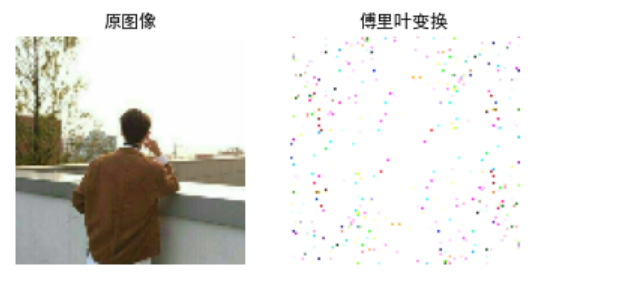上图是将原图进行缩放之后进行傅里叶变换的，只为了降低函数运行时间，尽快的得出结果；当然，OpenCV中也提供了图像傅里叶变换的库函数，接下，我们介绍OpenCV中傅里叶库函数的使用！

## 3、OpenCV库函数实现傅里叶变换

OpenCV 中相应的函数是cv2.dft()和用Numpy输出的结果一样，但是是双通道的。第一个通道是结果的实数部分，第二个通道是结果的虚数部分，并且输入图像要首先转换成 np.float32 格式。
1）、函数原型：img=cv2.dft(src, flags=None, nonzeroRows=None)

• src表示输入图像，需要通过np.float32转换格式
• flags表示转换标记，其中DFT _INVERSE执行反向一维或二维转换，而不是默认的正向转换；DFT _SCALE表示缩放结果，由阵列元素的数量除以它；DFT _ROWS执行正向或反向变换输入矩阵的每个单独的行，该标志可以同时转换多个矢量，并可用于减少开销以执行3D和更高维度的转换等；DFT _COMPLEX_OUTPUT执行1D或2D实数组的正向转换，这是最快的选择，默认功能；DFT _REAL_OUTPUT执行一维或二维复数阵列的逆变换，结果通常是相同大小的复数数组，但如果输入数组具有共轭复数对称性，则输出为真实数组
• nonzeroRows表示当参数不为零时，函数假定只有nonzeroRows输入数组的第一行（未设置）或者只有输出数组的第一个（设置）包含非零，因此函数可以处理其余的行更有效率，并节省一些时间；这种技术对计算阵列互相关或使用DFT卷积非常有用

注意，由于输出的频谱结果是一个复数，需要调用cv2.magnitude()函数将傅里叶变换的双通道结果转换为0到255的范围
2）、cv2.magnitude()函数原型：cv2.magnitude(x, y)

• x表示浮点型X坐标值，即实部
• y表示浮点型Y坐标值，即虚部

最终输出结果为幅值，即：

i m g ( I ) = x ( I ) 2 + y ( I ) 2 img(I)=\sqrt{x(I)^2+y(I)^2}
3）、傅里叶库函数使用

#傅里叶库函数使用
import cv2
import numpy as np
import matplotlib.pyplot as plt
plt.rcParams['font.sans-serif'] = ['SimHei'] #显示中文
#读取图像
#进行图像裁剪，加快傅里叶运算速率。将原始尺寸缩放为100*100的尺寸
#OpneCV傅里叶变换函数
result =cv2.dft(np.float32(img),flags = cv2.DFT_COMPLEX_OUTPUT)#需要将图像进行一次float转换
#将频谱低频从左上角移动至中心位置
dft_shift = np.fft.fftshift(result)
#频谱图像双通道复数转换为0-255区间
result1 = 20*np.log(cv2.magnitude(dft_shift[:,:,0], dft_shift[:,:,1]))
#图像显示
plt.subplot(121), plt.imshow(img,'gray'), plt.title('原图像')
plt.axis('off')
plt.subplot(122), plt.imshow(result1,'gray'), plt.title('傅里叶变换')
plt.axis('off')
plt.show()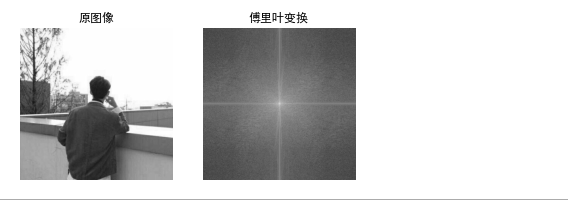使用OpenCV函数需要先将图像转为灰度图，然后才能进行处理，这里林君学长再介绍一个Numpy库的傅里叶变换处理，给出相关代码，小伙伴们自己理解哈，不做说明哦！
4）、扩展：Numpy库的图像傅里叶变换

#Numpy傅里叶变换
import cv2 as cv
import numpy as np
from matplotlib import pyplot as plt
plt.rcParams['font.sans-serif'] = ['SimHei'] #显示中文
#读取图像灰度图像
#快速傅里叶变换算法得到频率分布
f = np.fft.fft2(img)
#默认结果中心点位置是在左上角,
#调用fftshift()函数转移到中间位置
fshift = np.fft.fftshift(f)
#fft结果是复数, 其绝对值结果是振幅
fimg = np.log(np.abs(fshift))
#展示结果
plt.subplot(121), plt.imshow(img,'gray'), plt.title('原图像')
plt.axis('off')
plt.subplot(122), plt.imshow(fimg,'gray'), plt.title('傅里叶变换')
plt.axis('off')
plt.show()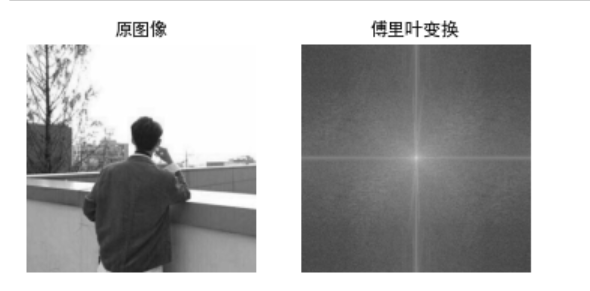# 二、傅里叶逆变换

## 1、傅里叶逆变换原理

1）、傅里叶反变换原理就是将图像频域还原为图像时域，具体原理公式如下所示：
f ( x , y ) = ∑ u = 0 H − 1 ∑ v = 0 W − 1 F ( u , v ) e 2 j π ( u x / H + v y / W ) 图 像 高 H ， 宽 W 。 f ( x , y ) 表 示 时 域 图 像 , F ( u , v ) 表 示 频 域 图 像 。 x 的 范 围 为 [ 0 , H − 1 ] ， y 的 范 围 为 [ 0 , W − 1 ] ↑ f(x,y)=\sum_{u=0}^{H-1}\sum_{v=0}^{W-1} F(u,v)e^{2j\pi(ux/H+vy/W)} \\\\ 图像高H，宽W。f(x,y)表示时域图像,F(u,v)表示频域图像。x的范围为[0,H-1]，y的范围为[0,W-1] ↑
对于公式的理解，和上面傅里叶变换公式理解一致

## 2、自定义傅里叶逆变换功能函数

1）、自定义傅里叶逆变换功能函数

import cv2 as cv
import numpy as np
from matplotlib import pyplot as plt
plt.rcParams['font.sans-serif'] = ['SimHei'] #显示中文
#傅里叶反变换
def idft(G):
H, W, channel = G.shape
#定义空白时域图像
out = np.zeros((H, W, channel), dtype=np.float32)
# 准备与原始图像位置相对应的处理索引
x = np.tile(np.arange(W), (H, 1))
y = np.arange(H).repeat(W).reshape(H, -1)
#通过公式遍历
for c in range(channel):
for u in range(H):
for v in range(W):
out[u, v, c] = np.abs(np.sum(G[..., c] * np.exp(2j * np.pi * (x * u / W + y * v / H)))) / np.sqrt(W * H)
# 剪裁
out = np.clip(out, 0, 255)
out = out.astype(np.uint8)
return out


2）、调用函数，先进行傅里叶变换，然后进行傅里叶逆变换并显示结果

#读取图像
#进行图像裁剪，加快傅里叶运算速率。将原始尺寸缩放为100*100的尺寸
img = cv2.resize(img, (100, 100), interpolation=cv2.INTER_CUBIC)
#BGR转换为RGB显示格式，方便通过matplotlib进行图像显示
img=cv2.cvtColor(img,cv2.COLOR_BGR2RGB)
#调用傅里叶变换函数,先将图像进行傅里叶变换，该函数上面我们通过原理写出了哦！
result =dft(img)
#将傅里叶频谱图从左上角移动到中心位置
fshift = np.fft.fftshift(result)
#将复数转为浮点数进行傅里叶频谱图显示
fimg = np.log(np.abs(fshift))
#调用傅里叶逆变换函数
result2=idft(result)
#图像显示
plt.subplot(131), plt.imshow(img), plt.title('原图像')
plt.axis('off')
plt.subplot(132), plt.imshow(fimg), plt.title('傅里叶变换')
plt.axis('off')
plt.subplot(133), plt.imshow(result2), plt.title('傅里叶逆变换')
plt.axis('off')
plt.show()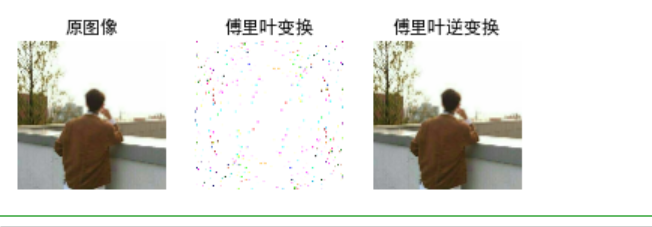最后我们可以看出，我们通过原理书写的傅里叶变换和傅里叶逆变换是没有问题的，因此，而且傅里叶反变换的结果是和之前的图像一模一样的，几乎没有什么损失，后面我们将会讲到在傅里叶变换的基础上实现高通或者说是低通滤波，来对图像去噪，记得收看啦！

## 3、OpenCV库函数实现傅里叶逆变换

OpenCV中也提供了傅里叶逆变换的库函数，我们一起看一下函数原型吧
1）、函数原型img=cv2.idft(src,flags,nonzeroRows)

• src表示输入图像，包括实数或复数
• flags表示转换标记
• nonzeroRows表示要处理的img行数，其余行的内容未定义

2）、OpenCV库函数实现傅里叶逆变换的完整代码如下所示：

#OpenCV实现傅里叶逆变换
import cv2 as cv
import numpy as np
from matplotlib import pyplot as plt
plt.rcParams['font.sans-serif'] = ['SimHei'] #显示中文

#读取图像
img = cv2.imread('my.jpg', 0)
#傅里叶变换
dft = cv2.dft(np.float32(img), flags = cv2.DFT_COMPLEX_OUTPUT)#傅里叶变换库函数调用
dftshift = np.fft.fftshift(dft)#将傅里叶频域从左上角移动到中间
res1= 20*np.log(cv2.magnitude(dftshift[:,:,0], dftshift[:,:,1]))#双通道结果转换为0到255的范围用于图像显示
#傅里叶逆变换
ishift = np.fft.ifftshift(dftshift)#将频域从中间移动到左上角
iimg = cv2.idft(ishift)#傅里叶逆变换库函数调用
res2 = cv2.magnitude(iimg[:,:,0], iimg[:,:,1])#双通道结果转换为0到255的范围
#显示图像
plt.subplot(131), plt.imshow(img, 'gray'), plt.title('原图像')
plt.axis('off')
plt.subplot(132), plt.imshow(res1, 'gray'), plt.title('傅里叶变换')
plt.axis('off')
plt.subplot(133), plt.imshow(res2, 'gray'), plt.title('傅里叶逆变换')
plt.axis('off')
plt.show()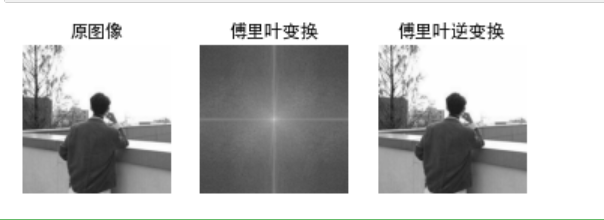3）、扩展：Numpy库也提供图像了傅里叶变换，完整代码如下所示：

#Numpy库的傅里叶变换
import cv2 as cv
import numpy as np
from matplotlib import pyplot as plt
plt.rcParams['font.sans-serif'] = ['SimHei'] #显示中文
#读取图像
#傅里叶变换
f = np.fft.fft2(img) #Numpy的库函数傅里叶变换
fshift = np.fft.fftshift(f)
res = np.log(np.abs(fshift))
#傅里叶逆变换
ishift = np.fft.ifftshift(fshift)
iimg = np.fft.ifft2(ishift) #Numpy的库函数傅里叶逆变换
iimg = np.abs(iimg)#将复数变为实数
#展示结果
plt.subplot(131), plt.imshow(img,'gray'), plt.title('原图像')
plt.axis('off')
plt.subplot(132), plt.imshow(res,'gray'), plt.title('傅里叶变换')
plt.axis('off')
plt.subplot(133), plt.imshow(iimg,'gray'), plt.title('Inverse Fourier Image')
plt.axis('off')
plt.show()以上就是本次博客的全部内容，遇到问题的小伙伴记得留言评论，学长看到会为大家进行解答的，这个学长不太冷！

任何的收获不是巧合，而是每天的努力与坚持得来的。人生因有梦想，而充满动力。
不怕你每天迈一小步，只怕你停滞不前；不怕你每天做一点事，只怕你无所事事。 坚持，是生命的一种毅力！执行，是努力的一种坚持！

陈一月的又一天编程岁月^ _ ^

展开全文计算机视觉 python opencv
• Opencv中使用的是dft函数，但是在很多场合需要纯C的代码，此程序完成了dft的函数功能，是纯C语言版本，只用了最基础的库。文件中有一幅lena的图像，将其归一化为32*32的大小，并将其像素值作为输入变量，输出结果为...
• 第14章：傅里叶变换一、理论基础：二、Numpy实现傅里叶变换：1. 实现傅里叶变换：2. 逆傅里叶变换：3. 高通滤波示例：三、OpenCV实现傅里叶变换：1. 实现傅里叶变换：2. 实现逆傅里叶变换：3. 低通滤波示例： 图像...

### 第14章：傅里叶变换

图像处理一般分为空间域处理和频率域处理。

空间域：

空间域处理是直接对图像内的像素点进行处理。空间域处理主要划分为灰度变换和空间滤波两种形式。灰度变换是对图像内的单个像素进行处理，比如调节对比度和处理域值等。空间滤波涉及图像的质量改变，比如图像平滑处理。空间域处理的计算简单方便，运算速度更快。

频率域：

频率域处理是先将图像变换的频率域，然后在频率域对图像进行处理，最后在通过反变换将图像从频率域变换到空间域。傅里叶变换是应用最广泛的一种频域变换，它能够将图像从空间域变换到频率域，而你傅里叶变换能够将频率域信息变换到空间域内。傅里叶变换在图像处理领域有着非常重要的作用。

下面从理论基础、基本实现、具体应用等角度对傅里叶变回进行简单的介绍。

## 一、理论基础：

傅里叶变换非常抽象，很多人在工程中用了很多年的傅里叶变换也没有彻底了解傅里叶变回到底是怎么回事。为了更好的说明傅里叶变换，我们先看生活中的一个例子。

下表所示是某饮料的配方，该配方是一个以时间形式表示的表格，表格很长，这里仅仅截取了其中一部分。该表中记录冲时刻“00：00”开始到某个特定时间“00:11”内的操作。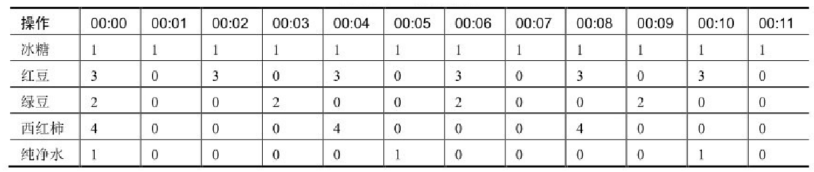分析表格发现，该配方：

• 每隔1分钟放一块冰糖。
• 每隔2分钟放3粒红豆。
• 每隔3分钟放2粒绿豆。
• 每隔4分钟放4块西红柿。
• 每隔5分钟放1杯纯净水。

上述文字是从操作频率的角度对配方进行说明。

在数据处理过程中，经常使用图表的形式表述信息。如果从时域的角度，该配方表可以表示为如下图形式。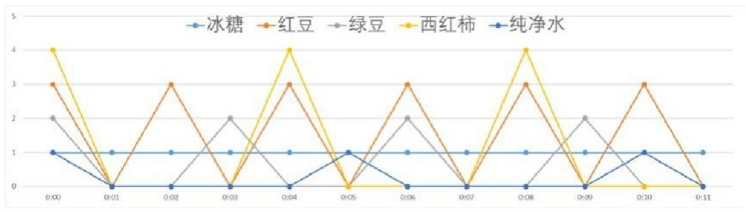如果从频率(周期)的角度表示，这个配方表可以表示为如下图形式，图中横坐标是周期(频率的倒数)，纵坐标是配料的份数。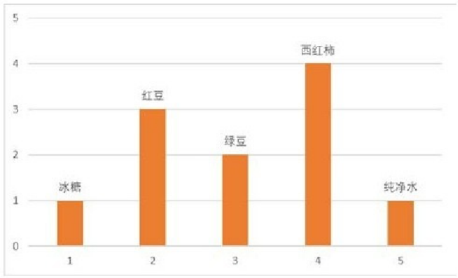对于函数，同样可以将其从时域变换到频域。下图是一个频率为5(1秒5个周期)、振幅为1的正弦曲线。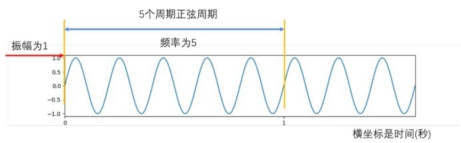如果从频率的角度考虑，则可以将其绘制为如下图所示的频域图。图中横坐标是频率，纵坐标是振幅。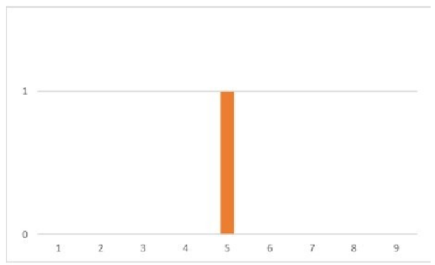上述两图是等价的，他们是一个函数的两种不同表示方式。可以通过频域表示得到对应的时域表示，也可以通过时域表示得到对应的频域表示。

法国数学家傅里叶指出，任何周期函数都可以表示为不同频率的正弦函数和的形式。在今天看来，这个理论是理所当然的，但是这个理论难以理解，在但是遭受了很大的质疑。

下面我们来看傅里叶变换的具体过程。例如，周期函数的曲线如下图左上角所示。该周期函数可以表示为：

• y = 3 * np.sin(0.8 * x) + 7 * np.sin(0.5 * x) +2 * np.sin(0.2 * x)

因此，该函数可以看成是由下列三个函数的和构成的：

• y1 = 3 * np.sin(0.8 * x)
• y2 = 7 * np.sin(0.5 * x)
• y3 = 2 * np.sin(0.2 * x)

上述三个函数对应的函数曲线分别如下图右上角、左下角、右下角的图所示。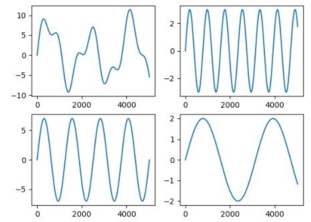如果从频域的角度考虑，上述三个正弦函数可以分别表示为下图中的三根柱子，图中横坐标是频率，纵坐标是振幅。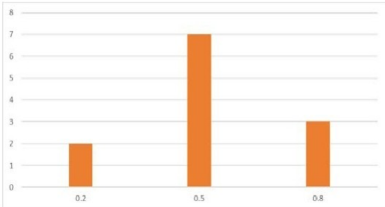通过以上分析可知，图中左上角的曲线可以表示为上图所示的频域图。

从左上角的时域函数图形，构造出上图所示频域图像的过程，就是傅里叶变换。

左上角的时域函数图形，与上图的频域图形表示的是完全相同的信息。傅里叶变换就是从频域的角度完整地表述时域信息。

除了上述的频率和振幅外，还要考虑时间的问题。例如，饮料配方为了控制风味，需要严格控制加入配料的时间。上表中“00:00”时刻的操作，在更精细的控制下，实际上如下表所示。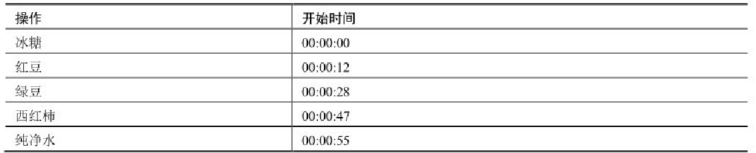如果加入配料的时间发生了变换，饮料的风味就会发生变化。所以，在实际处理过程中，还要考虑时间差。这个时间差，在傅里叶变换中就是相位。相位表述的时域时间差相关的信息。

例如，函数

• y = 3 * np.sin(0.8 * x) + 7 * np.sin(0.5 * 2 + 2) + 2 * np.sin(0.2 * x + 2)

可以看成下列三个函数的和的形式：

• y1 = 3 * np.sin(0.8 * x)
• y2 = 7 * np.sin(0.5 * x + 2)
• y3 = 2 * np.sin(0.2 * x + 3)

上述的四个函数分别对应的函数曲线为下图中的左上角、右上角、左下角、右下角。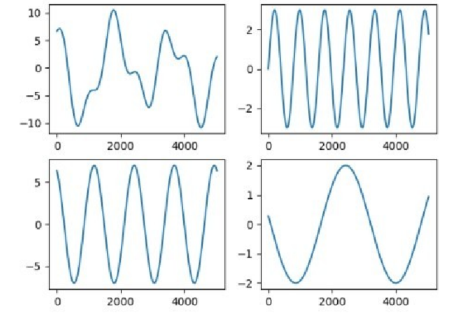​ 在本例中，如果把横坐标看成开始时间，则构成函数y的三个正弦函数并不都是从0时刻开始的，他们之间存在时间差。如果直接使用没有时间差的函数，则无法构成上图左上角所示的函数，而是会构成前面我们所说到的那个周期函数y = 3 * np.sin(0.8 * x) + 7 * np.sin(0.5 * x) +2 * np.sin(0.2 * x)。所以，相差是傅里叶变换中一个非常重要的条件。

​ 上面分别用饮料配方和函数的例子介绍了时域与频域转换的可行性，以帮助我们来理解傅里叶变换。

在图形处理过程中，傅里叶变换就是将图像分解成正弦分量和余弦分量两部分，即将图像从空间域转换到频率域。 数字图像经过傅里叶变换后，得到的频域是复数。因此，显示傅里叶变换的结果需要使用实数图像(real image)加虚数图像(complex image)，或者幅度图像(magnitude image)加相位图像(phase image)的形式。

​ 因为幅度图像中包含了原图我们所需要的大部分信息，所以图像处理过程中，通常仅使用幅度图像。当然，如果希望在频域内对图像进行处理，在通过逆傅里叶变换得到修改后的空域图像。就必须同时保留幅度图像和相位图像。

对图像进行傅里叶变换后，我们会得到图像中低频和高频的信息。低频信息对应图像中变化缓慢的灰度分量。高频信息对应图像内变换越来越快的灰度分量，是由灰度的尖锐过渡造成的。例如，在一幅大草原的图像中，低频信息就对应着广袤的颜色趋于一致的草原等细节信息，而高频信息则对应着狮子的轮廓等各种边缘及噪声信息。

傅里叶变换的目的，就是为了将图像从空域转换到频域，并在频域内实现对图像的特定处理，然后再对经过处理的频域图像进行逆傅里叶变换得到空域图像。 傅里叶变换在图像处理领域发挥着非常关键的作用，可以实现图像增强、图像去噪、边缘检测、特征提取、图像压缩和加密等。

## 二、Numpy实现傅里叶变换：

Numpy模块提供了傅里叶变换功能，Numpy模块中的fft2()函数可以实现图像的傅里叶变换。本节介绍如何用Numpy模块实现图像的傅里叶变换，以及在频域内过滤图像的低频信息，保留高频信息，实现高通滤波。

### 1. 实现傅里叶变换：

Numpy模块提供了实现傅里叶变换的函数numpy.fft.fft2()，它的语法格式是：

• 返回值=numpy.fft.fft2(原始图像)

这里需要注意的是，参数“原始图像”的类型是灰度图像，函数的返回值是一个复数数组(complex ndarray)。

​ 经过该函数的处理，就能得到图像的频谱信息。此时，图像频谱中的零频率分量位于频谱图像(频域图像)的左上角，为了便于观察，通常会使用numpy.fft.fftshift()函数将零频率成分移动到频域图像的中心位置，如图所示。函数numpy.fft.fftshift()的语法格式是：

• 返回值=numpy.fft.fftshift(原始频谱)

​ 使用该函数处理后，图像频谱中的零频率分量会被移到频域图像的中心位置，对于观察傅里叶变换后频谱中的零频率部分非常有效。

​ 对图像进行傅里叶变换后，得到的是一个复数数组。为了显示为图像，需要将它们的值调整到[0,255]的灰度空间内，使用的公式为：

• 像素新值=20*np.log(np.abs(频谱值))

示例1：实现傅里叶变换

import cv2
import numpy as np
import matplotlib.pyplot as plt

img = cv2.imread('../lena.bmp', 0)

f = np.fft.fft2(img)
fshift = np.fft.fftshift(f)
magnitude_spectrum = 20 * np.log(np.abs(fshift))

plt.subplot(1, 2, 1)
plt.imshow(img, cmap='gray')
plt.title('original')
plt.axis('off')

plt.subplot(1, 2, 2)
plt.imshow(magnitude_spectrum, cmap='gray')
plt.title('result')
plt.axis('off')
plt.show()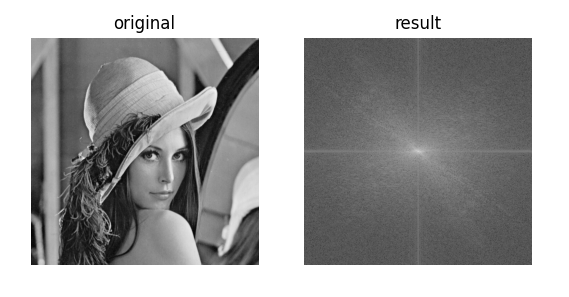### 2. 逆傅里叶变换：

​ 需要注意的是，如果在傅里叶变换过程中使用了numpy.fft.fftshift()函数移动零频率分量，那么在逆傅里叶变换过程中，需要先使用numpy.fft.ifftshift()函数将零频率分量移到原来的位置，再进行逆傅里叶变换。函数numpy.fft.ifftshift()是numpy.fft.fftshift()的逆函数，其语法格式为：

• 调整后的频谱=numpy.fft.ifftshift(原始频谱)

numpy.fft.ifft2()函数是numpy.fft.fft2()的逆函数。用来实现逆傅里叶变换，返回空域的复数数组。该函数的语法格式为：

• 返回值=numpy.fft.ifft2(频域数据)

函数numpy.fft.ifft2()的返回值仍旧是一个复数数组(complex ndarray)。

逆傅里叶变换得到的空域信息是一个复数数组，需要将该信息调整至[0,255]灰度空间内，使用的公式为：

• iimg=np.abs(逆傅里叶变换结果)

示例1：实现逆傅里叶变换

import cv2
import numpy as np
import matplotlib.pyplot as plt

img = cv2.imread('../boat.512.tiff', 0)
f = np.fft.fft2(img)
fshift = np.fft.fftshift(f)
magnitude_spectrum = 20 * np.log(np.abs(fshift))

ishift = np.fft.ifftshift(fshift)
iimg = np.fft.ifft2(ishift)
iimg = np.abs(iimg)

plt.subplot(131)
plt.imshow(img, cmap='gray')
plt.title('img')
plt.axis('off')

plt.subplot(132)
plt.imshow(magnitude_spectrum, cmap='gray')
plt.title('fft2')
plt.axis('off')

plt.subplot(133)
plt.imshow(iimg, cmap='gray')
plt.title('iimg')
plt.axis('off')
plt.show()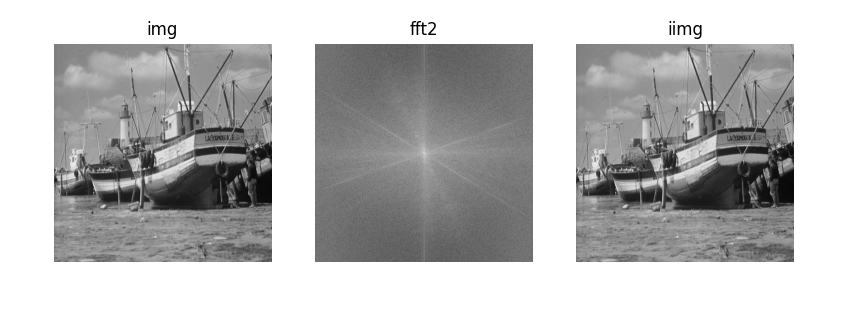### 3. 高通滤波示例：

在一幅图像内，同时存在着高频信号和低频信号。

• 低频信号对应图像内变化缓慢的灰度分量。例如，在一幅大草原的图像中，低频信号对应着颜色趋于一致的广袤草原。
• 高频信号对应图像内变化越来越快的灰度分量，是由灰度的尖锐过渡造成的。如果在上面的大草原图像中还有一头狮子，那么高频信号就对应着狮子的边缘等信息。

滤波器能够允许一定频率的分量通过或者拒绝其通过，按照其作用方式可以划分为低通滤波器和高通滤波器。

• 允许低频信号通过的滤波器称为低通滤波器。低通滤波器使高频信号衰减而对低频信号放行，会使图像变模糊。
• 允许高频信号通过的滤波器称为高通滤波器。高通滤波器使低频信号衰减而让高频信号通过，将增强图像中尖锐的细节，但是会导致图像的对比度降低。

傅里叶变换可以将图像的高频信号和低频信号分离。 例如，傅里叶变换可以将低频信号放置到傅里叶变换图像的中心位置，如前图所示，低频信号位于右图的中心位置。可以对傅里叶变换得到的高频信号和低频信号分别进行处理，例如高通滤波或者低通滤波。在对图像的高频或低频信号进行处理后，再进行逆傅里叶变换返回空域，就完成了对图像的频域处理。通过对图像的频域处理，可以实现图像增强、图像去噪、边缘检测、特征提取、压缩和加密等操作。

​ 例如，在下图所示，左图original是原始图像，中间的图像result是对左图original进行傅里叶变化后得到的结果，右图则是对result进行高通滤波后的结果。将傅里叶变换结果图像result中的低频分量值都替换为0(处理为黑色)，就屏蔽了低频信号，只保留高频信号，实现高通滤波。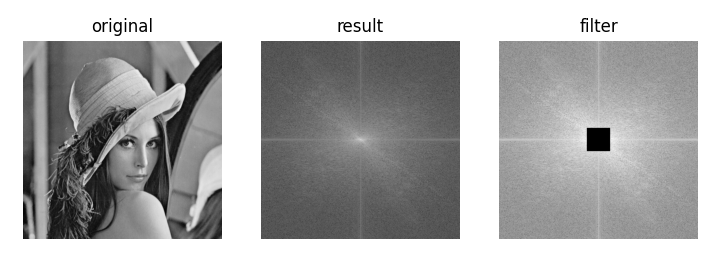要将上图中右图中间的像素值都置零，需要先计算其中心位置的坐标，然后选取以该坐标为中心，上下左右各30个像素大小的区域，将这个区域内的像素值置零。该滤波器的实现方法为：

• rows,cols=img.shape

crow,ccol=int(rows/2),int(cols/2)

fshift[crow-30:crow+30,ccol-30:ccol+30]=0

import cv2
import numpy as np
import matplotlib.pyplot as plt

img = cv2.imread('../boat.512.tiff', 0)

f = np.fft.fft2(img)
fshift = np.fft.fftshift(f)

rows, cols = img.shape
crow, ccol = int(rows / 2), int(cols / 2)
fshift[crow - 30: crow + 30, ccol - 30: ccol + 30] = 0

ishift = np.fft.ifftshift(fshift)
iimg = np.fft.ifft2(ishift)
iimg = np.abs(iimg)

plt.subplot(121)
plt.imshow(img, cmap='gray')
plt.title('original')
plt.axis('off')

plt.subplot(122)
plt.imshow(iimg, cmap='gray')
plt.title('iimg')
plt.axis('off')

plt.show()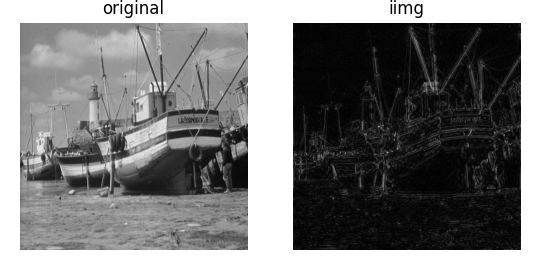## 三、OpenCV实现傅里叶变换：

OpenCV提供了函数cv2.dft()和cv2.idft()来实现傅里叶变换和逆傅里叶变换。

### 1. 实现傅里叶变换：

OpenCV中使用函数cv2.dft()进行傅里叶变换，语法格式为：

• 返回结果=cv2.dft(原始图像，转换标识)

在使用该函数时，需要注意参数的使用规范：

• 对于参数“原始图像”，要首先使用np.float32()函数将图像转换成np.float32格式。
• “转换标识”的值通常为“cv2.DFT_COMPLEX_OUTPUT”，用来输出一个复数阵列。

函数cv2.dft()返回的结果与使用Numpy进行傅里叶变换得到的结果是一致的，但是它返回的值是双通道的，第1个通道是结果的实数部分，第2个通道是结果的虚数部分。

​ 经过函数 cv2.dft()的变换后，我们得到了原始图像的频谱信息。此时，零频率分量并不在中心位置，为了处理方便需要将其移至中心位置，可以用函数numpy.fft.fftshift()实现。例如，如下语句将频谱图像 dft 中的零频率分量移到频谱中心，得到了零频率分量位于中心的频谱图像dftshift。

• dftShift=np.fft.fftshift(dft)

​ 经过上述处理后，频谱图像还只是一个由实部和虚部构成的值。要将其显示出来，还要做进一步的处理才行。

函数cv2.magnitude()可以计算频谱信息的幅度。该函数的语法格式为：

• 返回值=cv2.magnitude(参数1，参数2)
• 参数1：浮点型x坐标值，也就是实部。
• 参数2：浮点型y坐标值，也就是虚部，它必须和参数1具有相同的大小(size值的大小，不是value值的大小)。

函数cv2.magnitude()的返回值是参数1和参数2的平方和的平方根，公式为：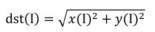I表示原始图像，dst表示目标图像。

得到频谱信息的幅度后，通常还要对幅度值做进一步的转换，以便将频谱信息以图像的形式展示出来。简单来说，就是需要将幅度值映射到灰度图像的灰度空间[0,255]内，使其以灰度图像的形式显示出来。

这里使用的公式为：

• result=20*np.log(cv2.magnitude(实部，虚部))

示例1：

import cv2
import numpy as np

img = cv2.imread('../lena.bmp', 0)
dft = cv2.dft(np.float32(img), flags=cv2.DFT_COMPLEX_OUTPUT)
dft_shift = np.fft.fftshift(dft)

result = 20 * np.log(cv2.magnitude(dft_shift[:, :, 0], dft_shift[:, :, 1]))

print(dft)
print(dft_shift)
print(result)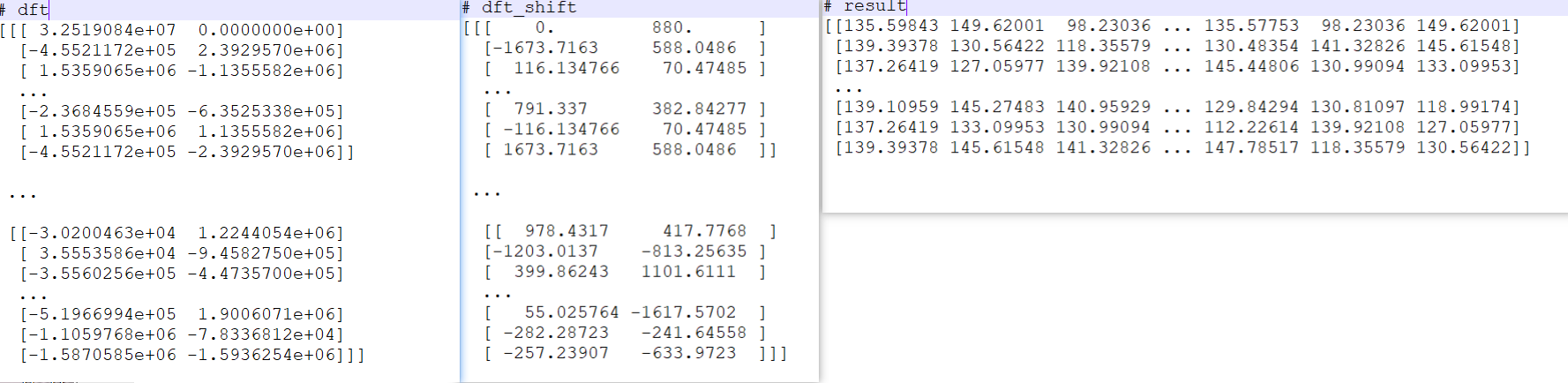示例2：用OpenCV函数对图像进行傅里叶变换，并展示其频谱信息。

import cv2
import numpy as np
import matplotlib.pyplot as plt

img = cv2.imread('../lena.bmp', 0)
dft = cv2.dft(np.float32(img), flags=cv2.DFT_COMPLEX_OUTPUT)
dft_shift = np.fft.fftshift(dft)
result = 20 * np.log(cv2.magnitude(dft_shift[:, :, 0], dft_shift[:, :, 1]))
plt.subplot(121)
plt.imshow(img, cmap='gray')
plt.title('original')
plt.axis('off')

plt.subplot(122)
plt.imshow(result, cmap='gray')
plt.title('result')
plt.axis('off')

plt.show()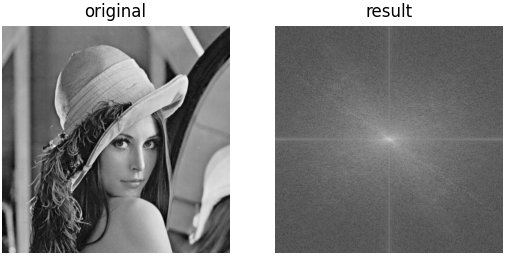### 2. 实现逆傅里叶变换：

在OpenCV中，使用函数cv2.idft()实现逆傅里叶变换，该函数是傅里叶变换函数cv2.dft()的逆函数。其语法格式为：

• 返回结果=cv2.idft(原始数据)

​ 对图像进行傅里叶变换后，通常会将零频率分量移至频谱图像的中心位置。如果使用函数numpy.fft.fftshift()移动了零频率分量，那么在进行逆傅里叶变换前，要使用函数numpy.fft.ifftshift()将零频率分量恢复到原来位置。

注意，在进行逆傅里叶变换后，得到的值仍旧是复数，需要使用函数cv2.magnitude()计算其幅度。

示例：

import cv2
import numpy as np
import matplotlib.pyplot as plt

img = cv2.imread('../boat.512.tiff', 0)
dft = cv2.dft(np.float32(img), flags=cv2.DFT_COMPLEX_OUTPUT)
dft_shift = np.fft.fftshift(dft)

rst = 20 * np.log(cv2.magnitude(dft_shift[:, :, 0], dft_shift[:, :, 1]))

ishift = np.fft.ifftshift(dft_shift)
iimg = cv2.idft(ishift)
iimg = cv2.magnitude(iimg[:, :, 0], iimg[:, :, 1])

plt.subplot(131)
plt.imshow(img, cmap='gray')
plt.title('original')
plt.axis('off')

plt.subplot(132)
plt.imshow(rst, cmap='gray')
plt.title('rst')
plt.axis('off')

plt.subplot(133)
plt.imshow(iimg, cmap='gray')
plt.title('iimg')
plt.axis('off')

plt.show()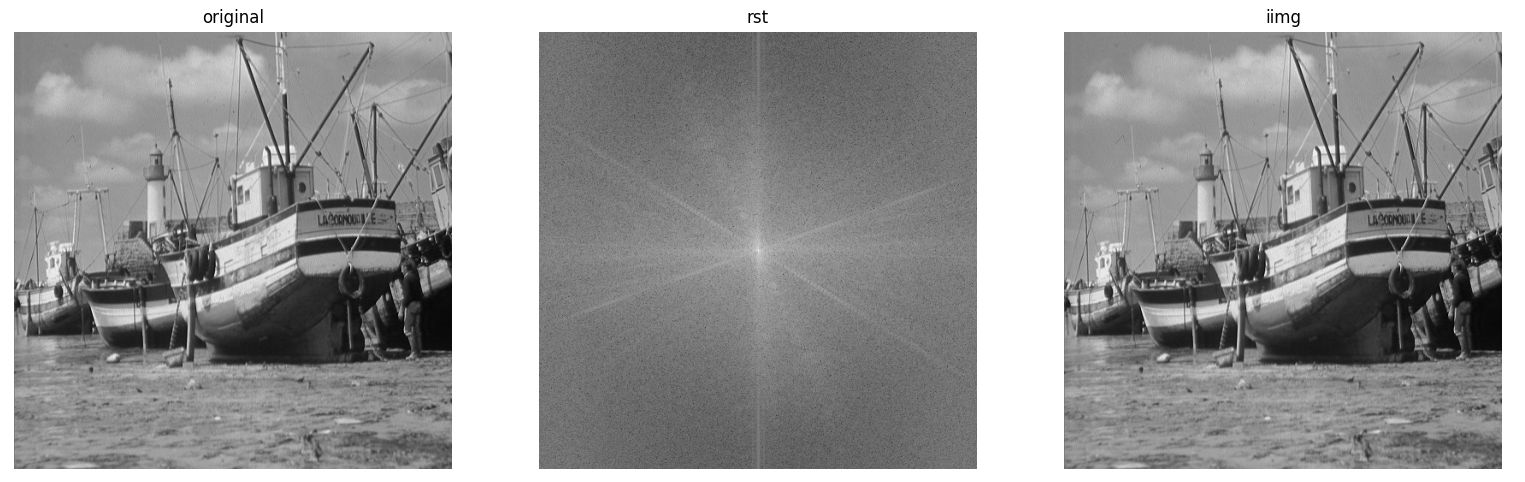### 3. 低通滤波示例：

​ 前面讲过，在一幅图像内，低频信号对应图像内变化缓慢的灰度分量。例如，在一幅大草原的图像中，低频信号对应着颜色趋于一致的广袤草原。低通滤波器让高频信号衰减而让低频信号通过，图像进行低通滤波后会变模糊。

​ 例如，在下图中，左图original是原始图像，中间的图像result是对original进行傅里叶变换后得到的结果，右图是低通滤波后的图像。将傅里叶变换结果图像result中的高频信号值都替换为0（处理为黑色），就屏蔽了高频信号，只保留低频信号，从而实现了低通滤波。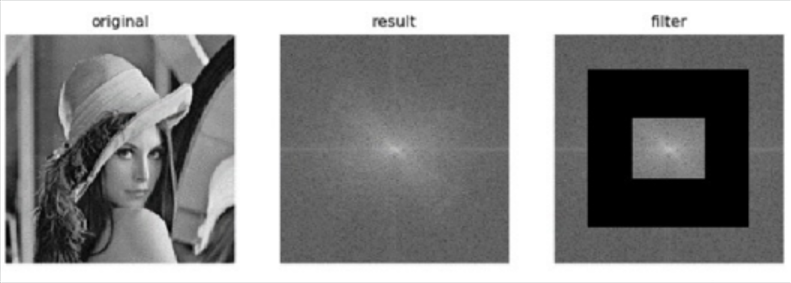在实现低通滤波时，可以专门构造一个如下图左图所示的掩码图像，用它与原图的傅里叶变换频谱图像进行与运算，就能将频谱图像中的高频信号过滤掉。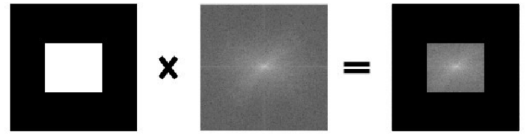import cv2
import numpy as np
import matplotlib.pyplot as plt

img = cv2.imread('../boat.512.tiff', 0)
dft = cv2.dft(np.float32(img), flags=cv2.DFT_COMPLEX_OUTPUT)
dft_shift = np.fft.fftshift(dft)

rows, cols = img.shape
crow, ccol = int(rows / 2), int(cols / 2)
mask = np.zeros((rows, cols, 2), np.uint8)
mask[crow - 30: crow + 30, ccol - 30: ccol + 30] = 1

f_shift = dft_shift * mask
print(f_shift)

# mask = np.zeros((rows, cols), np.uint8)
# mask[crow - 30: crow + 30, ccol - 30: ccol + 30] = 1
# print(f_shift)

ishift = np.fft.ifftshift(f_shift)
iimg = cv2.idft(ishift)
iimg = cv2.magnitude(iimg[:, :, 0], iimg[:, :, 1])

plt.subplot(121)
plt.imshow(img, cmap='gray')
plt.title('original')
plt.axis('off')

plt.subplot(122)
plt.imshow(iimg, cmap='gray')
plt.title('iimg')
plt.axis('off')

plt.show()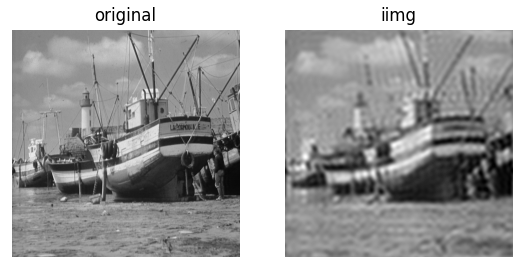展开全文计算机视觉 图像处理
• 即原始k点重排后位置： 即时域信号，x(n)，重排序号perm_num = mod([1:n]*sigma,n)+1，则对应重排后的傅里叶变换，频谱顺序与原信号频谱X，经过重排序号perm_num1 = mod([1:n]*inv_sigma,n)+1，位置始终相差1（数论...

作者：桂。

时间：2018-01-06  14:00:25

前言

一、稀疏FFT

主要是12年MIT的论文：Simple and Practical Algorithm for Sparse Fourier Transform。

核心思想：

SFT 作为这样一种“输出感知”算法,其核心思路是按照一定规则 Γ ( • )将信号频点投入到一组“筐”中(数量为 B，通过滤波器实现 ) . 因频域是稀疏的,各大值点将依很高的概率在各自的“筐”中孤立存在 . 将各“筐”中频点叠加,使 N 点长序列转换为 B点的短序列并作 FFT 运算,根据计算结果,忽略所有不含大值点的“筐”,最后根据对应分“筐”规则,设计重构算法 Γ-1 ( • )恢复出 N 点原始信号频谱。算法流程：步骤一：选定一个sigma，实现数据频域重排。

频域重排主要是因为FFT之后，频域大值集中在一起，需要将这一伙打散，保证频域的稀疏性(sparse)。打散之后就能稀疏，依据定理(可见n越大，打散的可能性越大，从这里看，sigma与B还是有关系的，sigma体现了相邻频点的最小间隔，而B决定了每个bucket的宽度)：这里是按一定概率(概率性)将频谱重排，MIT重排思路：对应代码：

fs = 1000;

f0 = 70;

N = 256;

t = [0:N-1]/fs;

x = exp(1j*2*pi*t*f0) ;

%%*************Step1:频谱随机重排**************

sigma = 19; %inv(sigma) = 27

tao = 3;

%permutation

perm_num = mod([1:N]*sigma+tao,N)+1;

pn = x(perm_num);

当然也有强制(确定性)将数据重排的思路，思路类似，只是实现方式不同：sigma的选择主要利用性质：这里sigma^-1是sigma关于模N的逆元(数论倒数)。该点主要说明：变化前后的完备性(信号可重建)。

步骤二：加窗。

这里根据筐的多少(B)，平分2pi频域，即带宽2pi/B，理想窗函数为矩形窗，但时域为无限长的sinc函数，需要加窗截断，可选择gausswin。

即win = sinc.*gausswin：这里不局限于gausswin，满足给定约束的窗函数均可：步骤三：频域抽取并作FFT

加窗之后，保证了频谱不至于展宽严重，进一步保证了稀疏性。频域降采样：等价于时域的混叠的傅里叶变换：故直接在时域进行处理。处理完成后进行FFT运算。

该操作的理论基础为：步骤四：哈希映射

哈希映射的线索为：最终观察的数据Y(k)【即y(n)】->P(k)【即p(n)】->X(k)【即x(n)】：

第一步(y -> p)转化的理论依据：第二步(p -> x)转化的理论依据：

序列重排后的频域变换为：这两步解释了算法step4的参数定义：但实际中并非第一步提到的理想情况，实际情况是：因此存在一个频谱重建的成功概率问题：4章节的后半部分主要在证明这个概率问题。

步骤五~六的主要目的，引用原文的说法：

Here are two versions of the inner loop: location loops and estimation loops. Location loops are given a parameter d, and output a set I ⊂ [n] of dkn/B  coordinates that contains each large coefficient with“good” probability. Estimation loops are given a set  I ⊂ [n] and estimate x I such that each coordinate is  estimated well with “good” probability.

步骤五：定位循环

d是一个参数，存在约束原文取值为：该步骤的主要操作为：

将 Z ( k )中 dK 个较大值(从大到小依次排列)的坐标( k)归入集合 J 中,通过哈希反映射得到 J 的原像：这样便得到包含原始频谱坐标的集合，迭代L次：迭代的目的？

步骤六：估值循环

对于每个k∈Ir，计算频率信息：步骤5~6主要操作：至此完成了稀疏FFT(sparse FFT)的整个运算过程，记作sfft_v01。

改进版都是依次大框架进行，不同点主要有三个方面：1)重排的实现思路；2)频谱的重建思路。不再一一展开。

原文示例：该示例给出了步骤5~6的直观解释：二、问题记录

仿真验证：n = 256点频信号，sigma = 19，则inv_sigma = 27，假设生成点频信号：图1为重排的信号，图2为重排加窗(此处窗不够理想，看到长尾，论文中强调加窗，作用在于把尾巴剁掉。)，图3为原始信号频谱，图4为降采样之后的频谱。可以看出重排容易引入谐波：以sigma为周期。

原始频谱位置为k=10-1 = 9(下标从0开始)，重排后对应频谱位置为mod(sigma*k,n) = 171 = 172-1，与理论分析一致。即原始k点重排后位置：即时域信号，x(n)，重排序号perm_num = mod([1:n]*sigma,n)+1，则对应重排后的傅里叶变换，频谱顺序与原信号频谱X，经过重排序号perm_num1 =  mod([1:n]*inv_sigma,n)+1，位置始终相差1(数论倒数的性质决定):mod(sigma*inv_sigma,n)=1

展开全文• 傅里叶变换的过程即是把这条周期曲线拆解成多个光滑正弦曲线的过程. 傅里叶变换的目的是将时域(时间域)上的信号转变为频域(频率域信号), 随着域的不同, 对一个事物的了解角度也会随之改变.因此某些在时域中不好处的...
• 程序完成傅里叶变换中图像的含义，详见维基百科：https://en.wikipedia.org/wiki/File:Fourier_series_square_wave_circles_animation.gif 程序中，circle控制圆的绘制，curve控制曲线的绘制，rotate控制圆的旋转。...
• 实验14 快速傅里叶变换(FFT)(完美格式版，本人自己完成，所有语句正确，不排除极个别错误，特别适用于山大，勿用冰点等工具下载，否则下载之后的word 格式会让很多部分格式错误，谢谢)XXXX 学号姓名处XXXX一、实验...
• 图6 2图像变换 2.1傅立叶变换 傅里叶变换可以将图像从空间域转换到频域，然后执行相应的处理. MATLAB中具有傅立叶变换功能. 原始图片: 图7 傅立叶变换 F=fft2(I1); S=abs(F); figure,imshow(S,[]); 效果: 图8 它...
• ## 快速傅里叶变换

千次阅读 2018-09-09 09:46:23
FFT，即为快速傅氏变换，是离散傅氏变换的快速算法，它是根据离散傅氏变换的奇、偶、虚、实等特性，对离散傅立叶变换的...我们可以这么理解：FFT（Fast Fourier Transformation）就是“快速傅里叶变换”的意思，它...傅里叶变换
• 本文是对斯坦福大学公开课-傅里叶变换及其应用的一些学习总结 对应的课程视频链接为：课程链接 课程用到的讲义下载地址：讲义链接 傅里叶变换的内容对应课程5-8节，讲义第二章。 由傅里叶级数到傅里叶变换 在...
• #include #include /*********************************************************************快速傅立叶变换函数简介：此函数是通用的快速傅里叶变换C 函数C 语言函数，移植性强，以下部分不依赖硬件。此函数采用...
• // 快速傅里 叶变换FFT的C语言算法...// 快速傅里叶变换（Fast Fourier Transform)是离散傅里叶变换的一种快速算法，简称FFT，通过FFT可以将一个信号从时域变换到频域。 // 模拟信号经过A/D转换变为数字信号的过程...快速傅里叶变换
• 作者：赵越链接：傅立叶变换，时域，频域 - 赵越的文章 - 知乎专栏来源：知乎著作权归作者所有。商业转载请联系作者获得授权，非商业转载请注明出处。目录信号分析方法概述时域频域时域与频域的互相转换傅立叶变换 ...
• matlab离散傅里叶变换平滑代码ECE 438：具有应用程序的数字信号处理 课程说明：本课程分为三个单元。 基础：连续时间和离散时间信号的回顾，以及频谱分析； 有限冲激响应和无限冲激响应数字滤波器的设计； 处理随机...
• 实验十二 离散傅里叶变换实验一、实验目的和要求二、实验内容三、实验仪器、设备四、实验原理五、实验步骤六、实验注意事项七、实验结果八、实验总结 一、实验目的和要求   理解傅立叶变换的基本原理；掌握实现傅...计算机视觉 opencv
• 将快速傅里叶变换公式用C语言编写出，实现输入序列完成对序列傅里叶变换功能，经过与matlab中的傅里叶变换进行对比证明，代码的精度符合一般要求
• }#include#include/********************************************************************* 快速傅立叶变换C程序包函数简介：此程序包是通用的快速傅里叶变换C语言函数，移植性强，以下部分不依 赖硬件。...
• 设N=2MN={{2}^{M}}N=2M，有限长序列x(n)x\left( n \right)x(n)的离散傅里叶变换(DFT)为 X(k)=∑n=0N−1x(n)e−jnk2πN,0≤k≤N−1X(k)=\sum\limits_{n=0}^{N-1}{x\left( n \right){{e}^{-jnk\frac{2\pi }{N}}}},0\le...
• 为什么要在频率域研究图像增强？ 利用频率成分和图像外表之间的对应关系。...傅里叶变换（Fourier Transform，FT）后，对同一事物的观看角度随之改变，可以从频域里发现一些从时域里不易察觉的特征。 原理：将满
• ## 傅里叶变换

千次阅读 2018-08-20 16:22:18
对于图像，2D离散傅里叶变换（DFT）用于找到频域．快速傅里叶变换（FFT）用于计算DFT． 对于正弦信号，x（t）= Asin（2πft），我们可以说f是信号的频率，如果采用其频域，我们可以看到f处的尖峰。 如果对信号进行...傅里叶变换
• ## Shor算法 or量子傅里叶变换？

千次阅读 多人点赞 2020-11-16 11:04:39
背景介绍与功能分析二. 算法流程 一. 背景介绍与功能分析 尽管我们都知道一个数，无论大小，都可以被分解成素数的乘积，也就是著名的质因数分解，但是对于较大位数的数字来说，能明确的找到质数因子却是一个非常...量子算法 机器学习
• 基于FPGA的快速傅里叶变换加速（三） 硬件加速介绍及部分verilog代码实现1. 硬件加速1.1 FPGA1.1.1 FPGA介绍概念：基本结构：工作原理：1.1.2 开发板开发板类型开发板介绍1.2 加速理念1.2.1 硬件加速1.2.2 FFT中的...
• 时域到频域的变换，从侧面可以看到频谱，这个频谱并没有包含时域中全部的信息，只代表每一个对应的正弦波的振幅。 1.2 相位谱   时域到频域的变换，我们得到了一个从侧面看的频谱，但是这个频谱并没有包含时域...
• 图像处理一般分为空间域处理和频率域处理。空间域处理是直接对图像额内部的...傅里叶变换是应用最广的一种频域变换，它能够将图像从空间域变换到频率域，同样，逆傅里叶变换则可以将频率域的信息变换到空间域内。 ...
• 万字长篇教你学会OpenCV快速傅里叶变换(FFT)用于图像和视频流的模糊检测python opencv
• 这不，无聊的假期里，我用它做了一个音频的频率计，通过电脑上的声卡采集声音，用傅里叶变换完成时域-频域的转换，最后确定声音的主频率。用这个简陋的频率计来给吉他定调，比专业的定音器还好玩。python 傅里叶变换 SciPy
• } /***************************************************************** 函数原型：void FFT(struct compx *xin) 函数功能：对输入的复数组进行快速傅里叶变换（FFT） 输入参数：*xin复数结构体组的首地址指针，......